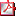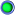Tech Log The very best in practical technical discussion on the web

# GroundRollCalculation

Thread Starter

Join Date: Jul 2013
Location: Moon
Posts: 4
GroundRollCalculation

Hello there,

I am trying to calculate the Ground Roll Distance for a Learjet 35, however I am having some difficulties getting to the correct result.

I attached a PDF with my calculation.

Maybe somone can find my mistake.

Thanks to all.
Patrick
Attached FilesGroundRollCalculation.pdf (117.7 KB, 37 views)Join Date: Nov 2010
Location: Atlanta
Age: 51
Posts: 341
Hello there,

I am trying to calculate the Ground Roll Distance for a Learjet 35, however I am having some difficulties getting to the correct result.

I attached a PDF with my calculation.

Maybe somone can find my mistake.

Thanks to all.
Patrick
I don't think I ever could, but out of curiosity, where did you find this formula?
And seeing as you have Vlof, wouldn't it be easier to assume constant acceleration?
F=M*a , so: 31.000 = 8200 * a , so a = 3.8m/s^2

t=(v2-v1)/a , so t = (73 - 0)/3.8 , so t = 19s
(142 kts = 73m/s)

s = 1/2at^2 , so s = 1/2 * 3.8 * 19^2 , so s = 685m or around 2,250 feet, acceleration probably isn't constant so your number could be right for a sea level departure at ISA on a Lear with all engines running.

Don't know where you get the 1700m from, but no-way you would take 1700m for ground roll a Lear. The numbers I see are 5,000ft so around 1700m for TODR, and that includes climbing to 50 feet with one engine failed at V1, at max gross.Thread Starter

Join Date: Jul 2013
Location: Moon
Posts: 4
I got the equation from a Bachelor Thesis. Unfortunately the professor does not have time to explain neither the equation nor my calculations to me. That is why I am asking here.
I am by no means capable of coming up with this equation on my own.
The 1700m are from flyAPG. But you are correct it is the TODR. So I figure from Lift Off to 35/50feet takes about 200m. So the ground roll is about 1500m, which at 18100 pounds seems realistic for me.Join Date: Jan 2004
Location: Hiding..... in one hemisphere or another
Posts: 999
Unfortunately the professor does not have time to explain neither the equation nor my calculations to me.
Wow, what an A\$\$hole!Join Date: Nov 2010
Location: Atlanta
Age: 51
Posts: 341
I got the equation from a Bachelor Thesis. Unfortunately the professor does not have time to explain neither the equation nor my calculations to me. That is why I am asking here.
I am by no means capable of coming up with this equation on my own.
The 1700m are from flyAPG. But you are correct it is the TODR. So I figure from Lift Off to 35/50feet takes about 200m. So the ground roll is about 1500m, which at 18100 pounds seems realistic for me.
I think the ratio for ground roll to TODR is probably closer to 2/3, so TODR 1700m, ground roll 1100m, but I am guessing here. I flew the CJ3, TODR is 3200ft, was almost always of the ground by 2000ft.

Honestly, I don't really understand the formula, but I will see how far I get:

The 1/2 and top of the equation is: 1/2 * m * v^2. This is the formula for kinetic energy.

The bottom of the equitation is T-D-F-Rwy slope.
So Thrust [N] - ("aerodynamic"(not really)) Drag [N] - (ground friction) Drag [N] - Loss/Gain of energy due to runway slope [N] , so basically the sum of all the horizontal forces or the net force on the aircraft.

Work is equal to the Force multiplied by the distance, so distance is Work divided by force.
The work done on an object by a net force equals the kinetic energy, so the distance traveled is the kinetic energy (top) divided by the total net force (bottom).

Where this equation doesn't make sense is that it puts the classic lift and drag formulas into a ground roll equation. Until you start your rotation the drag comes from 2 sources: the rolling drag from the wheels, and the aerodynamic drag from the whole aircraft. The D in the formula should be zero because it relates to induced drag associated with flying at an angle of attack that creates lift, and we really don't while rolling down the runway. I don't know where the friction coefficient of 0.04 comes from (scrambled eggs on a Teflon pan?), but I assume it is the friction coefficient for a tire on a runway, but we shouldn't subtract anything from it for lift until we rotate, so L should be zero until then. The part about runway slope could be correct. It uses v=.707vlof as an average speed but there is no L or D till rotation, and the formula has ZERO real aerodynamic drag in the equation.

I honestly think it isn't a time issue preventing your professor from explaining this equation, i think it is just plain incorrect, and unusable in any practical way.Join Date: Jul 2014
Location: Germany
Posts: 333
Drag as well as lift change with speed, so you can't possibly use one value for the speed in their formulas.

That formula looks like it's basically: s = 1/2 a t^2 with t = v/a
That only works for constant acceleration.

As i don't really have a clue myself i'll refer you to this page:
https://physics.stackexchange.com/qu...s-not-constant
To be specific your problem seems to be acceleration depending on velocity as described in case 3 here:
https://physics.stackexchange.com/a/15620

Now have fun with all those integrals.
(Because that's what you get when the acceleration isn't constant)Moderator

Join Date: Apr 2001
Location: various places .....
Posts: 6,495
The following link is to Sydney Uni's aero eng course work. The authors are pretty serious academic researchers and know their stuff. Worth a read for those who are interested in the engineering basics of this and that to do with flying machines. (Not that I'd give a plug to a particular university .. even if my engineering degree is from there ..)

Aerodynamics for Students | www.aerodynamics4students.com

The specific discussion on takeoff and landing is at this link -

http://www.aerodynamics4students.com...nd-landing.phpJoin Date: Feb 2019
Location: shiny side up
Posts: 382
Lear 35 AFM...enjoy!You may not post new threads
You may not post replies
You may not post attachments
You may not edit your posts

BB code is On
Smilies are On
[IMG] code is On
HTML code is Off
Trackbacks are Off
Pingbacks are Off
Refbacks are Off

Thread Tools
Search this ThreadShow Printable VersionEmail this Page
Search this Thread:

Advanced Search

Copyright © 2018 MH Sub I, LLC dba Internet Brands. All rights reserved. Use of this site indicates your consent to the Terms of Use.